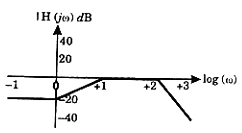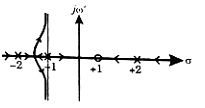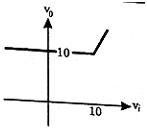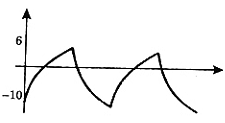# The Bode magnitude plot of H(jω) = (104(1 + jω))/((10 + jω)(100 + jω)2) is

1.

2.

3.

4.

4Explanation :
No Explanation available for this question

# A closed-loop system has the characteristic function (s2 - 4) (s + 1) + K (s - 1) = 0. Its root locus plot against K is

1.

2.

3.

4.

4Explanation :
No Explanation available for this question

# The algebraic equation F(s) = s5 - 3s4 + 5s3 - 7s2 + 4s + 20 is given. F(s) = 0 has

1.  a single complex root with the remaining roots being real

2.  one positive real root and four complex roots, all with positive real parts

3.  one negative real root, two imaginary roots, and two roots with positive real arts

4.  one positive real root, two imaginary roots, and two roots with negative real parts

4

one negative real root, two imaginary roots, and two roots with positive real arts

Explanation :
No Explanation available for this question

# Consider the following Nyquist plots of loop transfer functions over ω = 0 to ω = ∞.Which of these plots represents a stable closed loop system

1.  (1) only

2.  all, except (1)

3.  all, except (3)

4.   (1) and (2) only

4

(1) only

Explanation :
No Explanation available for this question

# A current of - 8 + 6√(2) (sin ωt + 30°) A is passed through three meters. They are a centre zero PMMC meter, a true rms meter and a moving iron instrument. The respective readings (in A) will be

1.  8, 6, 10

2.  8, 6, 8

3.  -8, 10, 10

4.  -8, 2, 2

4

-8, 10, 10

Explanation :
No Explanation available for this question

# A 200/1 Current transformer (CT) is wound with 200 turns on the secondary on a toroidal core. When it carries a current of 160 A on the primary, the ratio and phase errors of the CT are found to be -0.5% and 30 minutes respectively. If the number of secondary turns is reduced by 1 the new ratio error (%) and phase error (min) will be respectively

1.  0.0, 30

2.  -0.5, 35

3.  -1.0, 30

4.  -1.0, 25

4

0.0, 30

Explanation :
No Explanation available for this question

# R1 and R4 are the opposite arms of a Wheatstone bridge as are R3 and R2. The source voltage is applied across R1 and R3. Under balanced conditions which one of the following is true

1.  R1 = R3 R4 / R2

2.  R1 = R2 R3 / R4

3.  R1 = R2 R4 / R3

4.  R1 = R2 + R3 + R4

4

R1 = R2 R3 / R4

Explanation :
No Explanation available for this question

# Assuming the diodes D1 and D2 of the circuit shown in figure to be ideal ones, the transfer characteristics of the circuit will be

1.

2.

3.

4.

4Explanation :
No Explanation available for this question

# Consider the circuit shown in figure. If the β of the transistor is 30 and ICBO is 20 nA and the input voltage is +5 V, then transistor would be operating in

1.  saturation region

2.  active region

3.  breakdown region

4.   cut-off region

4

saturation region

Explanation :
No Explanation available for this question

# A relaxation oscillator is made using OPAMP as shown in figure. The supply voltages of the OPAMP are ± 12V. The voltage waveform at point P will be

1.

2.

3.

4.

4